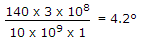# Electronics and Communication Engineering - Microwave Communication

### Exercise :: Microwave Communication - Section 1

21.

Which of the following parameters is negligible in transmission lines?

 A. R B. L C. C D. G

Explanation:

Shunt conductance can be neglected in most of calculations.

22.

The width of a radio beam from a 1 m diameter parabolic antenna at 10 GHz is about

 A. 100° B. 50° C. 5° D. 1°

Explanation:.

23.

The diagram to show distance time history of electrons in klystron amplifier is called

 A. apple gate diagram B. asynchronous diagram C. bunching diagram D. velocity modulation diagram

Explanation:

Applegate diagram is distance time plot.

24.

Impedance level of Impatt diodes is generally lower than that of Gunn diodes

 A. True B. False

Explanation:

An Impatt diode has n+ - p - i - p + structure and is used with reverse bias.

It exhibits negative resistance and operates on the principle of avalanche breakdown.

Impatt diode circuits are classified as broadly tunable circuit, low Q circuit and high Q circuit.

The impedance of Impatt diode is a few ohms. The word Impatt stands for Impact Avalanche Transit Time diode.

The features of Impatt diode oscillator are : frequency 1 to 300 GHz, Power output (0.5 W to 5 W for single diode circuit and upto 40 W for combination of several diodes), efficiency about 20%.

Its applications include police radar systems, low power microwave transmitter etc.

25.

A quarter wave line open circuited at far end behaves as

 A. inductance B. L and C in parallel C. capacitance D. L and C in series